Triply charged uranium-235 and uranium-238 ions are being separated in a mass spectrometer. (The much rarer uranium-235 is used as reactor f

Question

Triply charged uranium-235 and uranium-238 ions are being separated in a mass spectrometer. (The much rarer uranium-235 is used as reactor fuel.) The masses of the ions are 3.90×10−25kg3.90×10−25kg and 3.95×10−25kg,3.95×10−25kg, respectively, and they travel at 3.0×105m/s3.0×105m/s in a 0.250-T field. What is the separation between their paths when they hit a target after traversing a semicircle? (b) Discuss whether this distance between their paths seems to be big enough to be practical in the separation of uranium-235 from uranium-238.

in progress 0
5 months 2021-09-03T06:11:04+00:00 1 Answers 2 views 0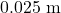Yes

Explanation: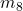= Mass of uranium 238 ion =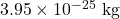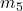= Mass of uranium 235 ion =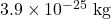v = Velocity of ions =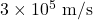q = Charge of triply charged ions =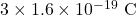B = Magnetic field = 0.25 T

The force balance is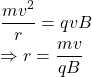The difference between the radius of the ions are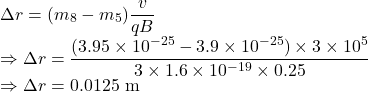Separation is given by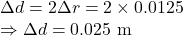The separation between their paths when they hit a target after traversing a semicircle is.

Yes, the distance between the paths is 2.5 cm is a practical separation between as it is easily measurable.